# 人工智能之Numpy与Pandas培训文档

pandas使用matplatlib进行数据库可视化展示的。使用pandas将数据分析后的结果用图表展示出来。

``In : import matplotlib.pyplot as plt``

``In : %matplotlib inline``

Pandas的Series和DataFrame中的plot()方法内部是用plt.plot()进行绘图的。

``````In : ts = pd.Series(np.random.randn(1000), index=pd.date_range('1/1/2000', periods=1000))

In : ts = ts.cumsum() # 求累和

In : ts.plot() # 画图
Out: <matplotlib.axes._subplots.AxesSubplot at 0x7f20d5690710>``````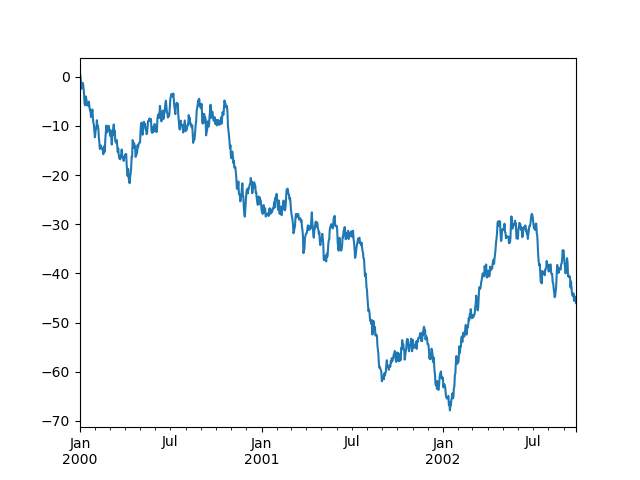``````In : df = pd.DataFrame(np.random.randn(1000, 4), index=ts.index, columns=list('ABCD'))

In : df = df.cumsum()

In : plt.figure(); df.plot();``````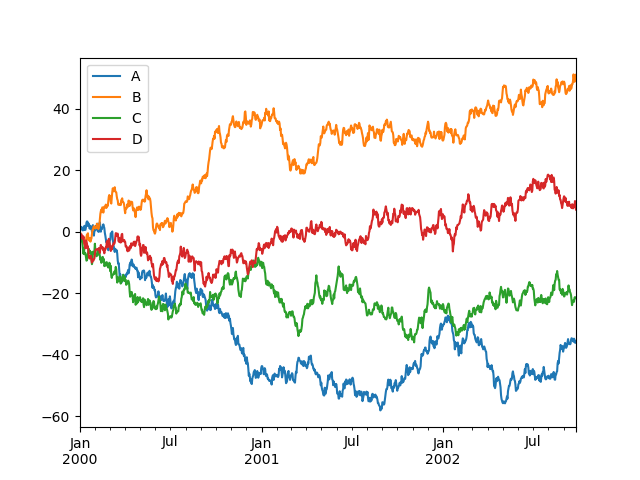``````In : df3 = pd.DataFrame(np.random.randn(1000, 2), columns=['B', 'C']).cumsum()

In : df3['A'] = pd.Series(list(range(len(df))))

In : df3.plot(x='A', y='B') # x轴标签为A，y轴标签为B
Out: <matplotlib.axes._subplots.AxesSubplot at 0x7f20d5aabf28>``````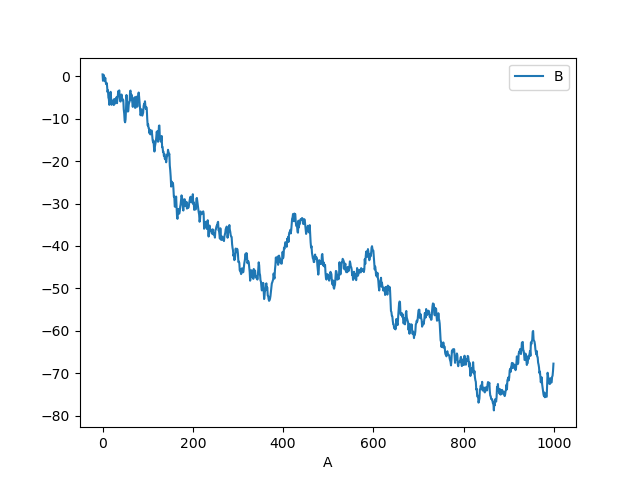``````In : plt.figure();

In : df.iloc.plot(kind='bar');``````

``````In : df = pd.DataFrame()

In : df.plot.<TAB>
df.plot.area     df.plot.barh     df.plot.density  df.plot.hist     df.plot.line     df.plot.scatter
df.plot.bar      df.plot.box      df.plot.hexbin   df.plot.kde      df.plot.pie``````

<TAB>表示按直tab键自动提示。

``````In : plt.figure();

In : df.iloc.plot.bar(); plt.axhline(0, color='k')
Out: <matplotlib.lines.Line2D at 0x7f20d46a4358>``````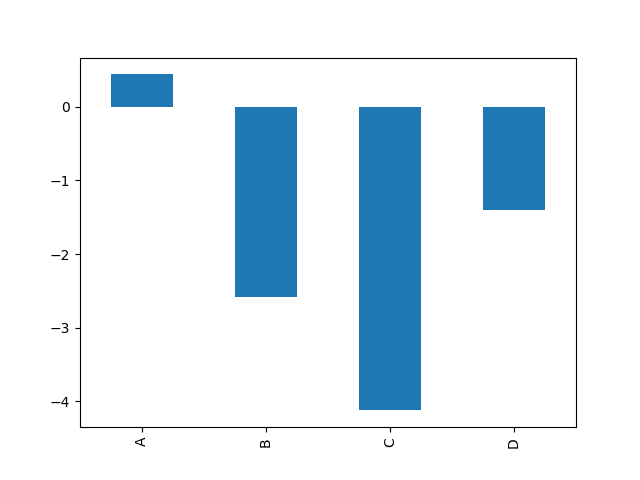``````In : df2 = pd.DataFrame(np.random.rand(10, 4), columns=['a', 'b', 'c', 'd'])

In : df2.plot.bar();``````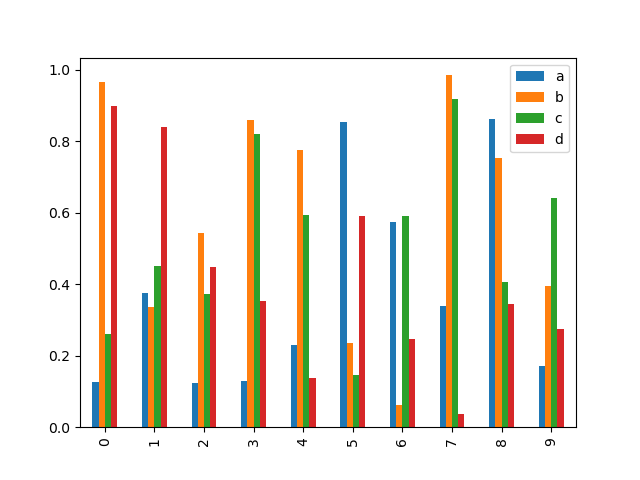``In : df2.plot.bar(stacked=True);``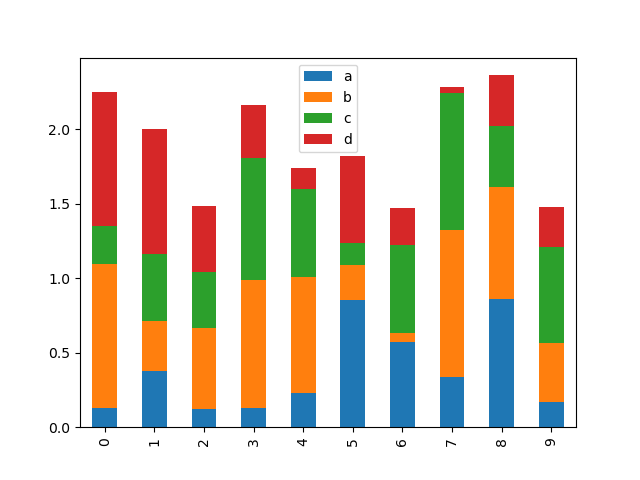``In : df2.plot.barh(stacked=True);``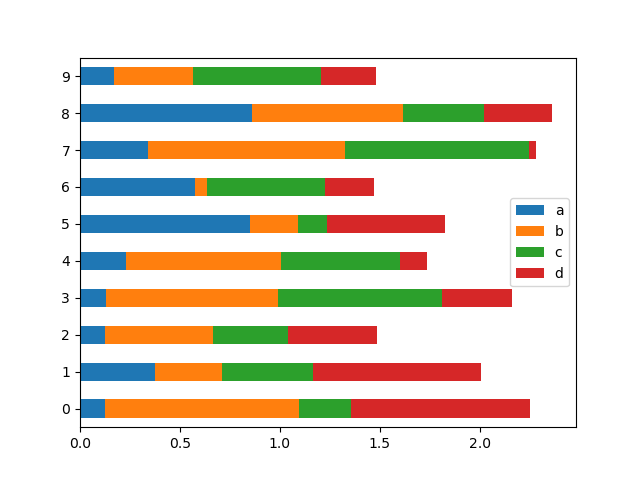``````In : df4 = pd.DataFrame({'a': np.random.randn(1000) + 1, 'b': np.random.randn(1000),
....:                     'c': np.random.randn(1000) - 1}, columns=['a', 'b', 'c'])
....:

In : plt.figure();

In : df4.plot.hist(alpha=0.5)
Out: <matplotlib.axes._subplots.AxesSubplot at 0x7f20cf918908>``````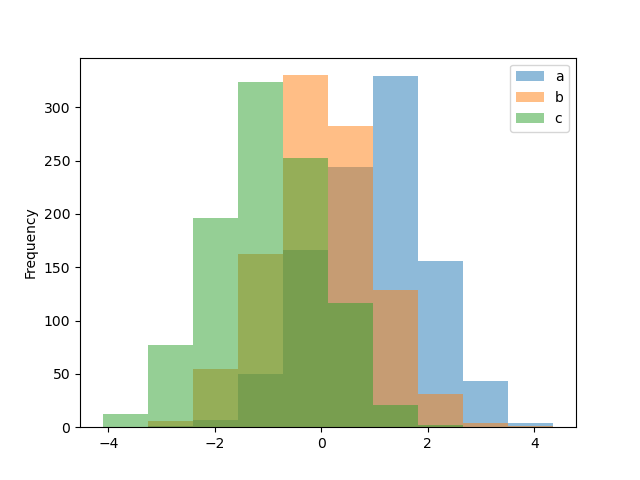``````In : plt.figure();

In : df4.plot.hist(stacked=True, bins=20)
Out: <matplotlib.axes._subplots.AxesSubplot at 0x7f20cf909a58>``````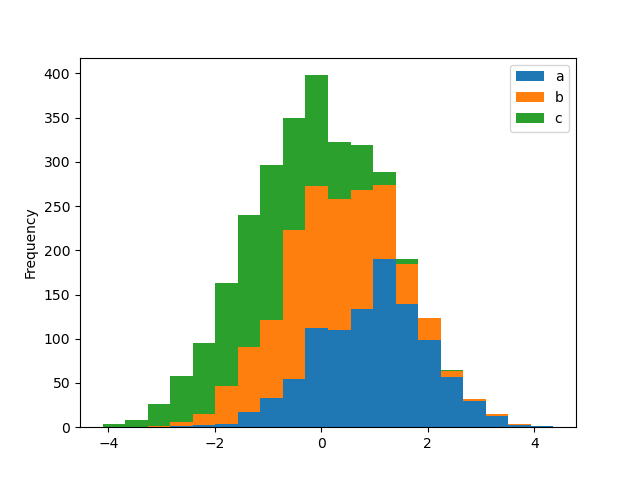``````In : plt.figure();

In : df4['a'].plot.hist(orientation='horizontal', cumulative=True)
Out: <matplotlib.axes._subplots.AxesSubplot at 0x7f20cfbceba8>``````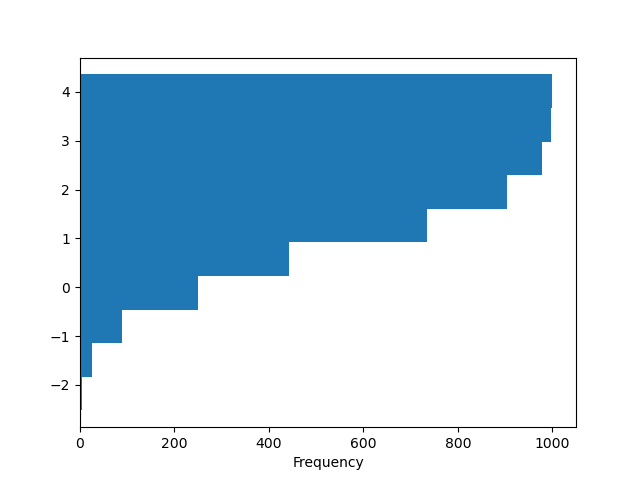``````In : plt.figure();

In : df['A'].diff().hist()
Out: <matplotlib.axes._subplots.AxesSubplot at 0x7f20d550efd0>``````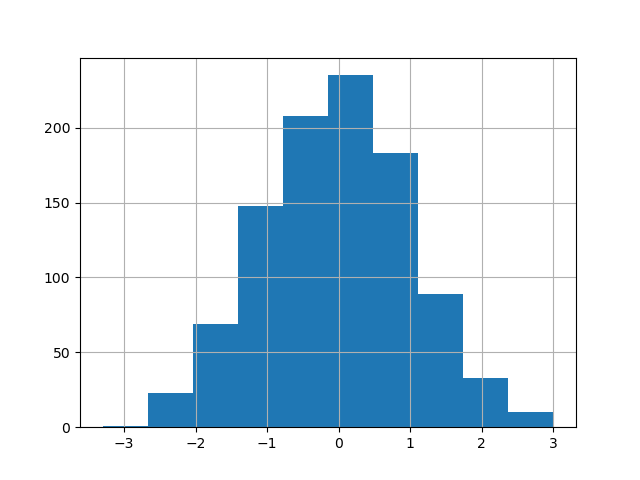DataFrame.hist()绘制多个子图上列的直方图：

``````In : plt.figure()
Out: <Figure size 640x480 with 0 Axes>

In : df.diff().hist(color='k', alpha=0.5, bins=50)
Out:
array([[<matplotlib.axes._subplots.AxesSubplot object at 0x7f20d47d7b38>,
<matplotlib.axes._subplots.AxesSubplot object at 0x7f20d490e978>],
[<matplotlib.axes._subplots.AxesSubplot object at 0x7f20d4924c88>,
<matplotlib.axes._subplots.AxesSubplot object at 0x7f20d41aff98>]], dtype=object)``````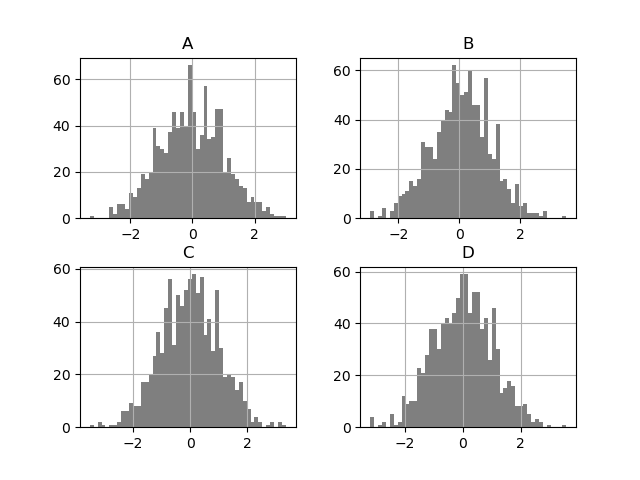``````In : data = pd.Series(np.random.randn(1000))

In : data.hist(by=np.random.randint(0, 4, 1000), figsize=(6, 4))
Out:
array([[<matplotlib.axes._subplots.AxesSubplot object at 0x7f20d51d9e48>,
<matplotlib.axes._subplots.AxesSubplot object at 0x7f20d5763c50>],
[<matplotlib.axes._subplots.AxesSubplot object at 0x7f20d576af60>,
<matplotlib.axes._subplots.AxesSubplot object at 0x7f20d5a012b0>]], dtype=object)``````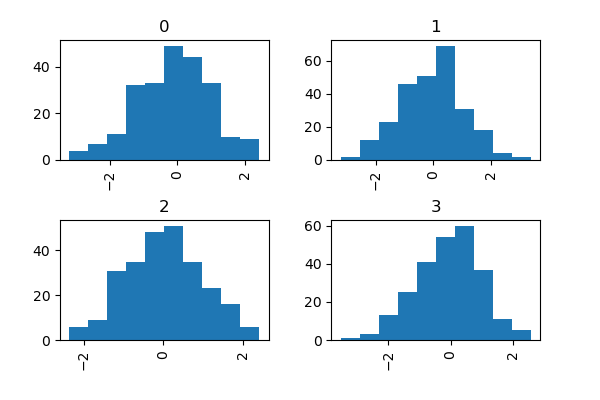``````In : df = pd.DataFrame(np.random.rand(10, 5), columns=['A', 'B', 'C', 'D', 'E'])

In : df.plot.box()
Out: <matplotlib.axes._subplots.AxesSubplot at 0x7f20cf9400f0>``````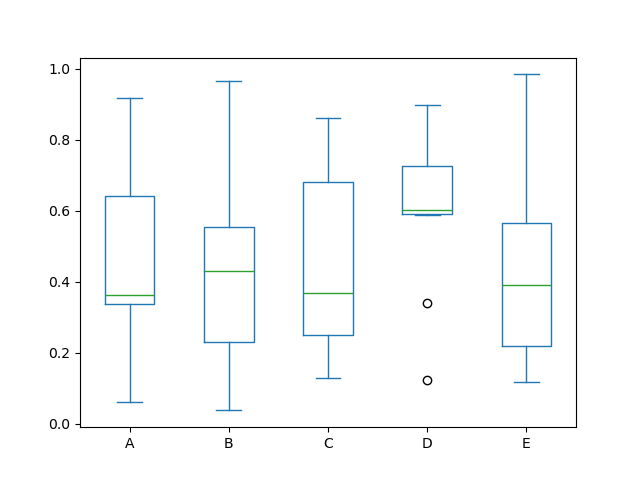Boxplot可以通过color参数来指定显示的图形颜色，你可以传递键为`boxes``whiskers``medians和``caps的字典。如果字典中缺失了某些值，就使用黙认的颜色。boxplot也可以通过指sym参数来指定传单风格。`

`当您通过color关键字传递其他类型的参数时，它将直接传递给matplotlib中，为boxs``whiskers``medians` 和`caps进行着色。`

`color应用于要绘制的每个框。 如果您想要更复杂的着色，可以通过传递return_type来获取每个绘制的艺术。`

``````In : color = dict(boxes='DarkGreen', whiskers='DarkOrange',
....:              medians='DarkBlue', caps='Gray')
....:

In : df.plot.box(color=color, sym='r+')
Out: <matplotlib.axes._subplots.AxesSubplot at 0x7f20d5907828>````````````In : df.plot.box(vert=False, positions=[1, 4, 5, 6, 8])
Out: <matplotlib.axes._subplots.AxesSubplot at 0x7f20d5be8198>``````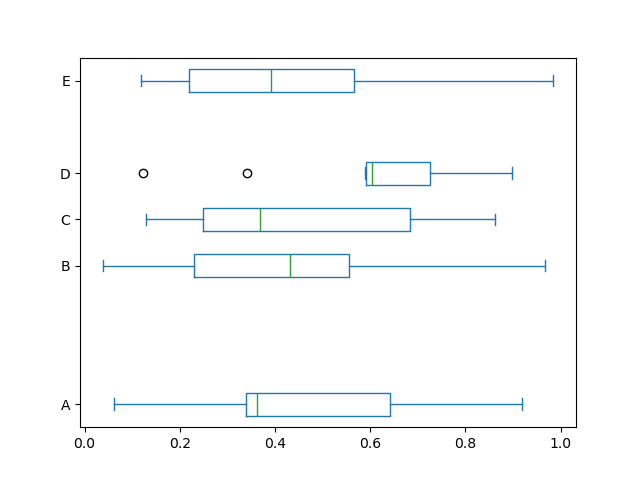``````In : df = pd.DataFrame(np.random.rand(10,5))

In : plt.figure();

In : bp = df.boxplot()``````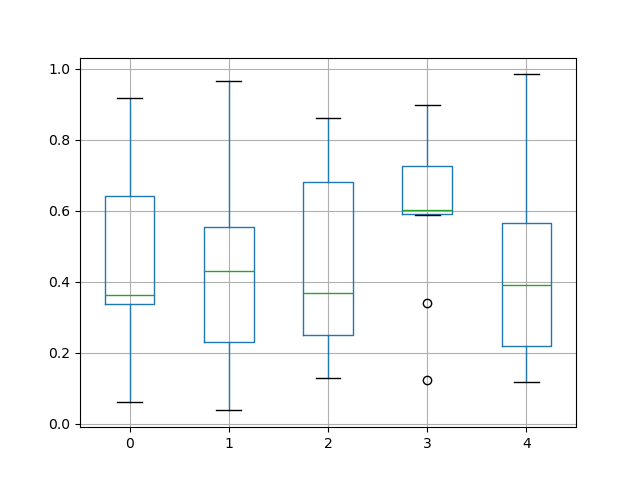``````In : df = pd.DataFrame(np.random.rand(10,2), columns=['Col1', 'Col2'] )

In : df['X'] = pd.Series(['A','A','A','A','A','B','B','B','B','B'])

In : plt.figure();

In : bp = df.boxplot(by='X')``````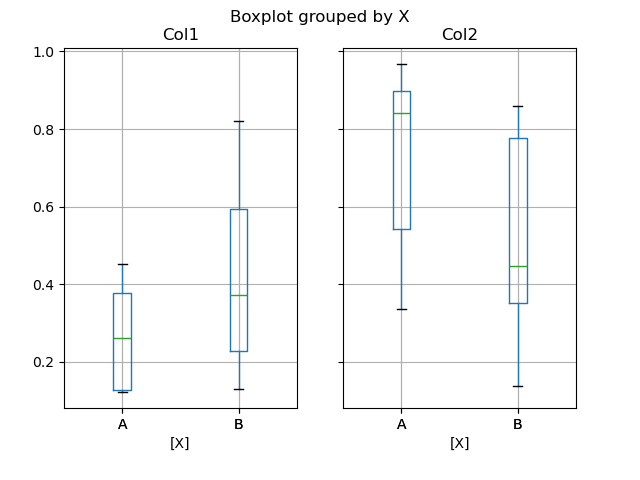``````In : df = pd.DataFrame(np.random.rand(10,3), columns=['Col1', 'Col2', 'Col3'])

In : df['X'] = pd.Series(['A','A','A','A','A','B','B','B','B','B'])

In : df['Y'] = pd.Series(['A','B','A','B','A','B','A','B','A','B'])

In : plt.figure();

In : bp = df.boxplot(column=['Col1','Col2'], by=['X','Y'])``````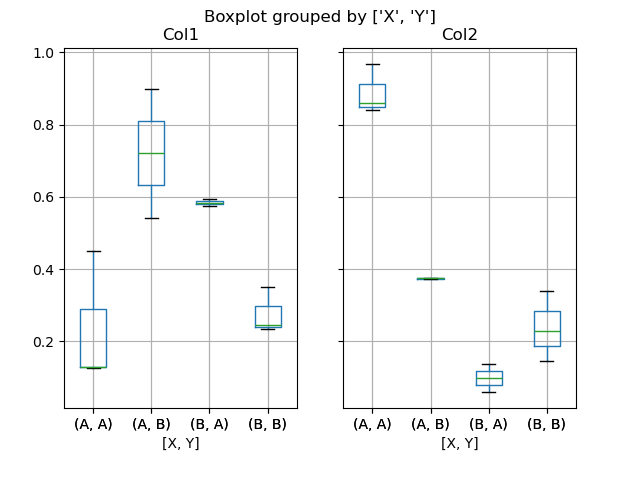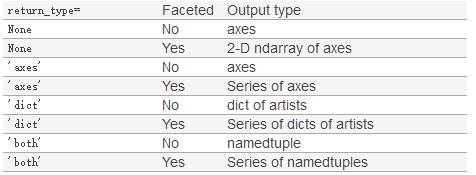Groupby.boxplot始终返回Series类型。

``````In : np.random.seed(1234)

In : df_box = pd.DataFrame(np.random.randn(50, 2))

In : df_box['g'] = np.random.choice(['A', 'B'], size=50)

In : df_box.loc[df_box['g'] == 'B', 1] += 3

In : bp = df_box.boxplot(by='g')``````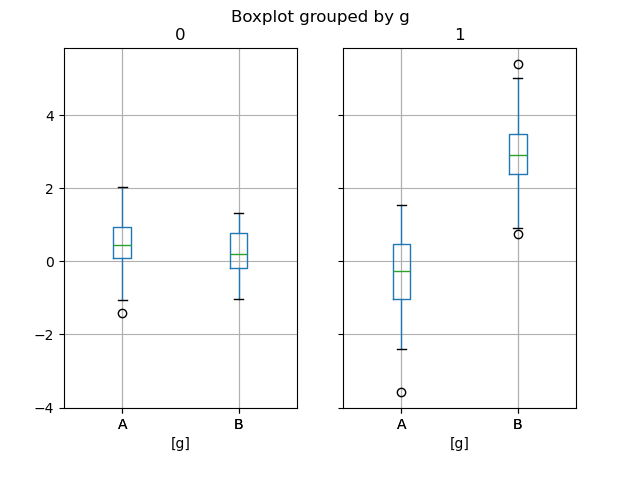``In : bp = df_box.groupby('g').boxplot()``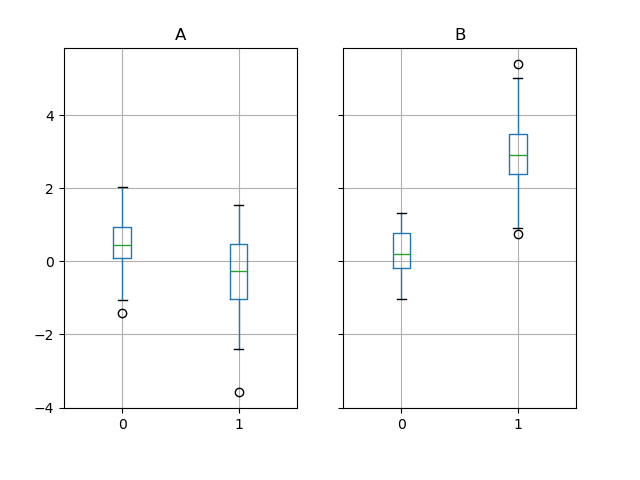``````In : df = pd.DataFrame(np.random.rand(10, 4), columns=['a', 'b', 'c', 'd'])

In : df.plot.area();``````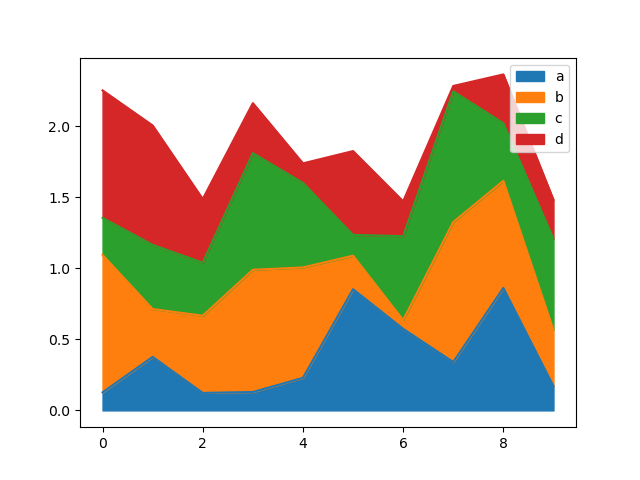``In : df.plot.area(stacked=False);``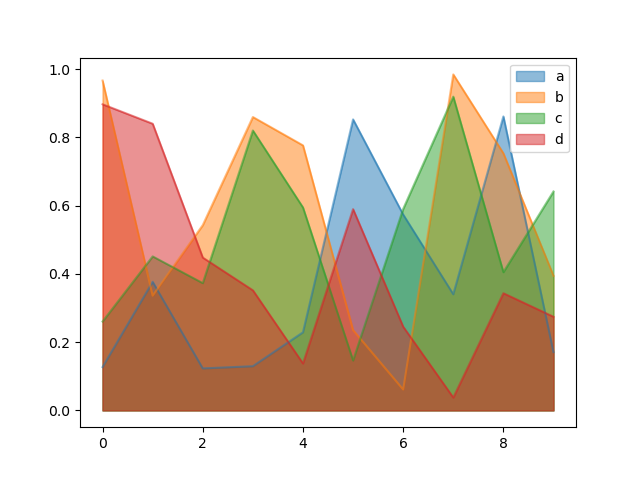``````In : df = pd.DataFrame(np.random.rand(50, 4), columns=['a', 'b', 'c', 'd'])

In : df.plot.scatter(x='a', y='b');``````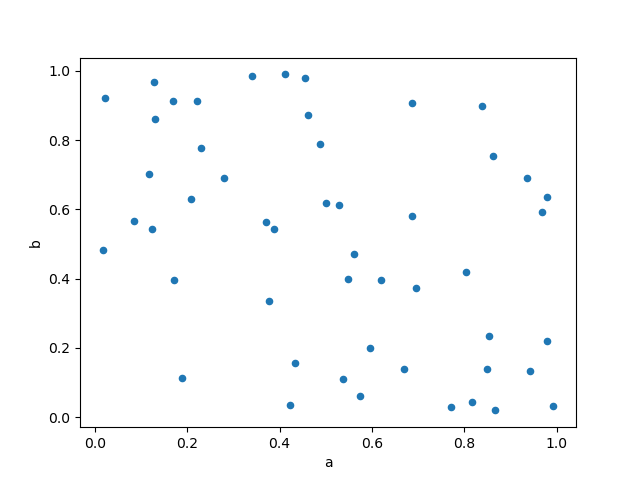``````In : ax = df.plot.scatter(x='a', y='b', color='DarkBlue', label='Group 1');

In : df.plot.scatter(x='c', y='d', color='DarkGreen', label='Group 2', ax=ax);``````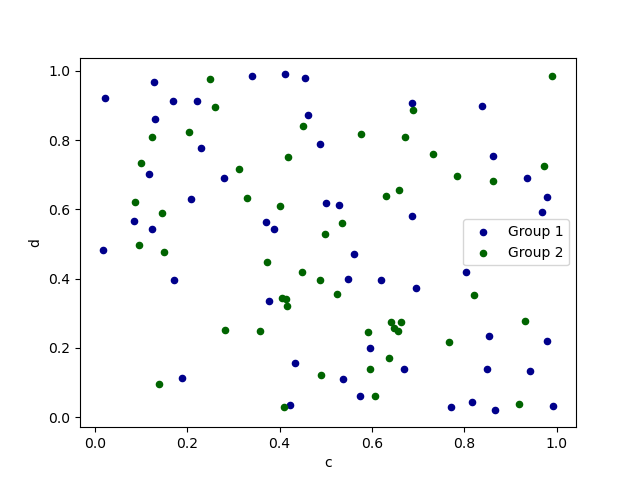``In : df.plot.scatter(x='a', y='b', c='c', s=50);``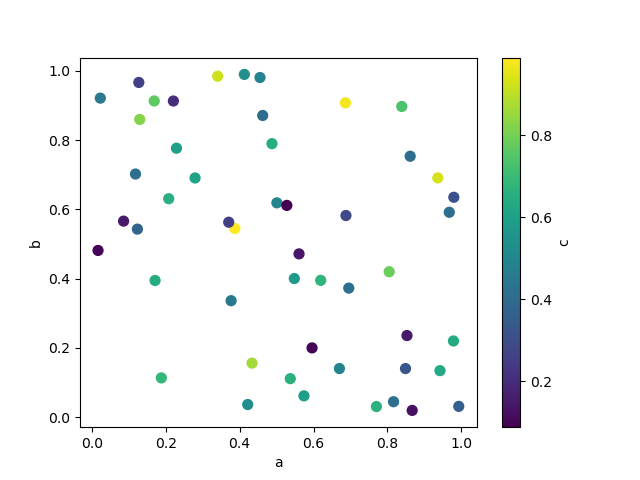``In : df.plot.scatter(x='a', y='b', s=df['c']*200);``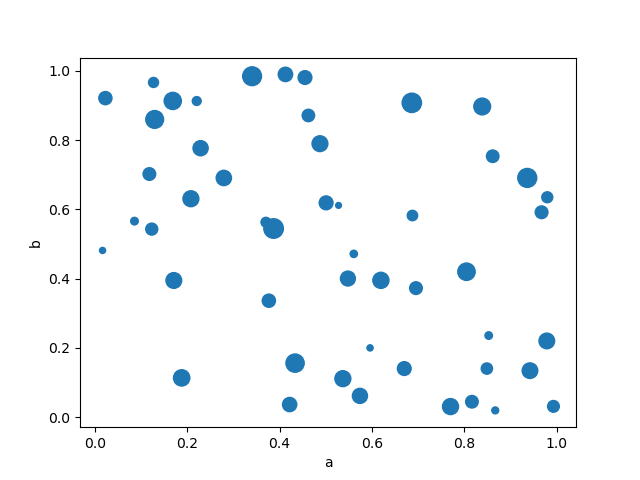``````In : df = pd.DataFrame(np.random.randn(1000, 2), columns=['a', 'b'])

In : df['b'] = df['b'] + np.arange(1000)

In : df.plot.hexbin(x='a', y='b', gridsize=25)
Out: <matplotlib.axes._subplots.AxesSubplot at 0x7f20c0e53b70>``````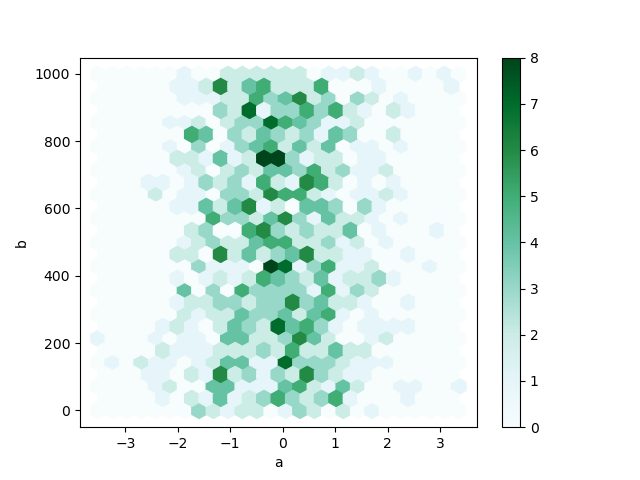``````In : df = pd.DataFrame(np.random.randn(1000, 2), columns=['a', 'b'])

In : df['b'] = df['b'] = df['b'] + np.arange(1000)

In : df['z'] = np.random.uniform(0, 3, 1000)

In : df.plot.hexbin(x='a', y='b', C='z', reduce_C_function=np.max,
....:         gridsize=25)
....:
Out: <matplotlib.axes._subplots.AxesSubplot at 0x7f20c0df8f98>``````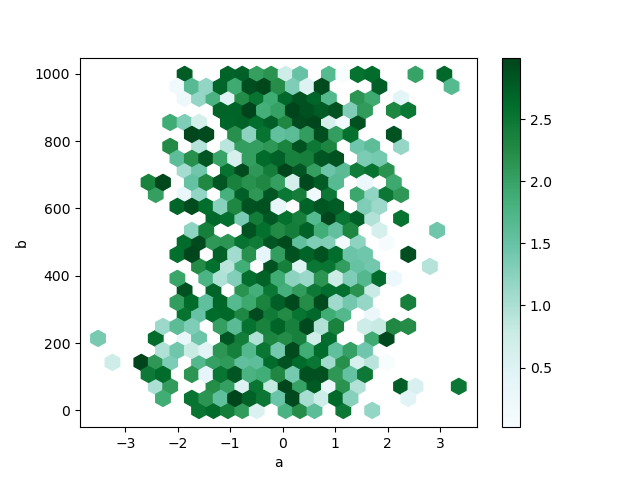``````In : series = pd.Series(3 * np.random.rand(4), index=['a', 'b', 'c', 'd'], name='series')

In : series.plot.pie(figsize=(6, 6))
Out: <matplotlib.axes._subplots.AxesSubplot at 0x7f20c0d15908>``````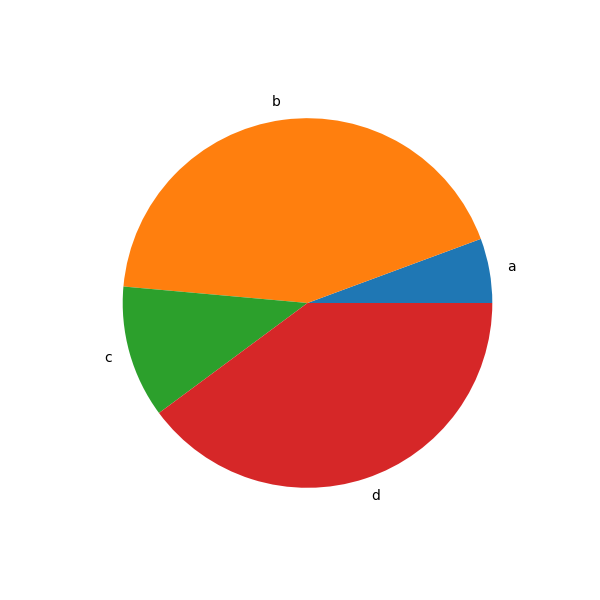``````In : df = pd.DataFrame(3 * np.random.rand(4, 2), index=['a', 'b', 'c', 'd'], columns=['x', 'y'])

In : df.plot.pie(subplots=True, figsize=(8, 4))
Out:
array([<matplotlib.axes._subplots.AxesSubplot object at 0x7f20c0cfdb00>,
<matplotlib.axes._subplots.AxesSubplot object at 0x7f20c0ca3ef0>], dtype=object)``````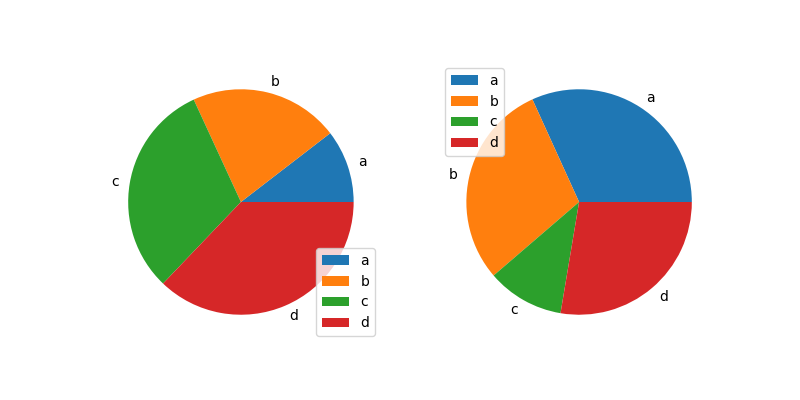``````In : series.plot.pie(labels=['AA', 'BB', 'CC', 'DD'], colors=['r', 'g', 'b', 'c'],
....:                 autopct='%.2f', fontsize=20, figsize=(6, 6))
....:
Out: <matplotlib.axes._subplots.AxesSubplot at 0x7f20c0c25668>``````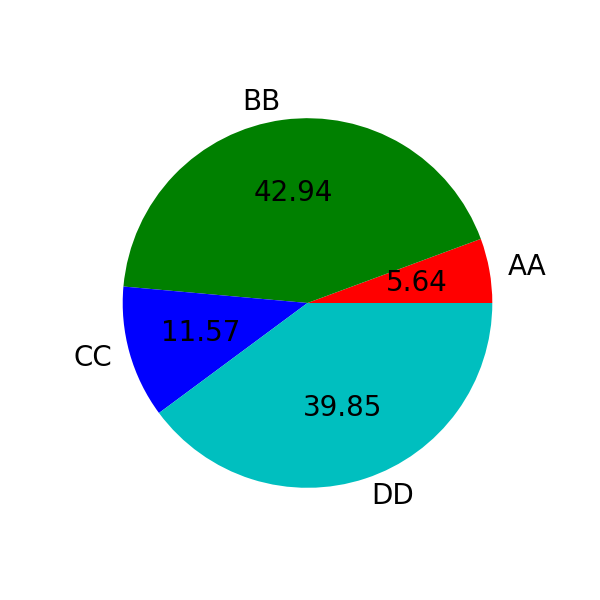https://matplotlib.org/users/index.html

Python就是个工具，知道它是干什么的？知道它有哪些库？分别用来做什么？numpy和pandas是怎么来分析数据的，大家只要知道有哪些分析函数？用来做什么样的运算？具体细节没必要知道，只要选几个函数或方法理解pandas的分析原理即可，都搞会了也没用，一个月就忘记了，是不是？